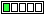All about flooble | fun stuff | Get a free chatterbox | Free JavaScript | Avatarsperplexus dot infoGiven one, get the other three (Posted on 2017-12-18)A 2-digit number, divisible by 11, is multiplied by 99.

If the 3rd digit is 7, what is the 4-digit product?

 No Solution Yet Submitted by Ady TZIDON Rating: 3.0000 (1 votes)Comments: ( Back to comment list | You must be logged in to post comments.)Analytical solution Comment 2 of 2 |Multiplying by 99 is the same as multiplying by 100 and then subtracting the original number.

If the original number is kk, then after multiplying by 99 the product equals

kk00
-  kk

This is a 4 digit number, whose digits (in order) are

k, k-1, 9-k, and 10-k

If 9-k = 7 then k = 2 and the digits are 2,1,7 and 8.

 Posted by Steve Herman on 2017-12-19 09:05:56Please log in:

 Search: Search body:
Forums (0)